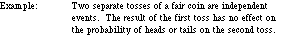index: click on a letter A B C D E F G H I J K L M N O P Q R S T U V W X Y Z A to Z index index: subject areas numbers & symbols sets, logic, proofs geometry algebra trigonometry advanced algebra & pre-calculus calculus advanced topics probability & statistics real world applications multimedia entrieswww.mathwords.com about mathwords website feedback

 Independent Events Events for which the probability of any one event occurring is unaffected by the occurrence or non-occurrence of any of the other events. Formally, A and B are independent if and only if P(A|B) = P(A).See also